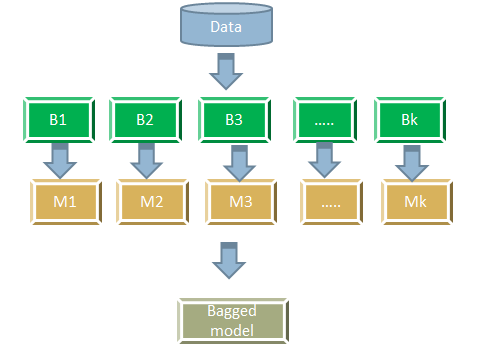• No products in the cart.

# 204.7.4 The Bagging Algorithm

##### The Bagging Algorithm.

Link to the previous post : https://statinfer.com/204-7-3-types-of-ensemble-models/

Let’s, move forward to the first type of Ensemble Methodology, the Bagging Algorithm.

We will cover the concept behind Bagging and implement it using python.

### The Bagging Algorithm

• The training dataset D
• Draw k boot strap sample sets from dataset D
• For each boot strap sample i
• Build a classifier model Mi
• We will have total of k classifiers M1,M2,...Mk
• Vote over for the final classifier output and take the average for regression output.### Why Bagging Works

• We are selecting records one-at-a-time, returning each selected record back in the population, giving it a chance to be selected again.
• Note that the variance in the consolidated prediction is reduced, if we have independent samples. That way we can reduce the unavoidable errors made by the single model.
• In a given boot strap sample, some observations have chance to select multiple times and some observations might not have selected at all.
• There a proven theory that boot strap samples have only 63% of overall population and rest 37% is not present.
• So the data used in each of these models is not exactly same, This makes our learning models independent. This helps our predictors have the uncorrelated errors..
• Finally the errors from the individual models cancel out and give us a better ensemble model with higher accuracy.
• Bagging is really useful when there is lot of variance in our data.

And now, lets put everything into practice.

### Practice : Bagging Models

• Import Boston house price data.
• Get some basic meta details of the data
• Take 90% data use it for training and take rest 10% as holdout data
• Build a single linear regression model on the training data.
• On the hold out data, calculate the error (squared deviation) for the regression model.
• Build the regression model using bagging technique. Build at least 25 models
• On the hold out data, calculate the error (squared deviation) for the consolidated bagged regression model.
• What is the improvement of the bagged model when compared with the single model?
In :
#Importing Boston house price data
import pandas as pd
import sklearn as sk
import numpy as np
import scipy as sp

In :
house.head(5)

Out:
crim zn indus chas nox rm age dis rad tax ptratio black lstat medv
0 0.00632 18.0 2.31 0 0.538 6.575 65.2 4.0900 1 296 15.3 396.90 4.98 24.0
1 0.02731 0.0 7.07 0 0.469 6.421 78.9 4.9671 2 242 17.8 396.90 9.14 21.6
2 0.02729 0.0 7.07 0 0.469 7.185 61.1 4.9671 2 242 17.8 392.83 4.03 34.7
3 0.03237 0.0 2.18 0 0.458 6.998 45.8 6.0622 3 222 18.7 394.63 2.94 33.4
4 0.06905 0.0 2.18 0 0.458 7.147 54.2 6.0622 3 222 18.7 396.90 5.33 36.2
In :
###columns of the dataset##
house.info()

<class 'pandas.core.frame.DataFrame'>
RangeIndex: 506 entries, 0 to 505
Data columns (total 14 columns):
crim       506 non-null float64
zn         506 non-null float64
indus      506 non-null float64
chas       506 non-null int64
nox        506 non-null float64
rm         506 non-null float64
age        506 non-null float64
dis        506 non-null float64
tax        506 non-null int64
ptratio    506 non-null float64
black      506 non-null float64
lstat      506 non-null float64
medv       506 non-null float64
dtypes: float64(11), int64(3)
memory usage: 55.4 KB

In :
###Splitting the dataset into training and testing datasets
from sklearn.cross_validation import train_test_split
house_train,house_test=train_test_split(house,train_size=0.9)

In :
###Building a linear Regression with medv as the predictor variable on the traiing dadaset ###
from sklearn.linear_model import LinearRegression
lr = LinearRegression()

Out:
LinearRegression(copy_X=True, fit_intercept=True, n_jobs=1, normalize=False)
In :
###predicting the model on test dataset

In :
from sklearn.metrics import mean_squared_error

###error in linear regression model ###
mean_squared_error(house_test['medv'],predict_test, sample_weight=None, multioutput='uniform_average')

Out:
22.567044241536465
In :
#Build the regression model using bagging technique.
from sklearn.ensemble import BaggingRegressor
from sklearn.linear_model import LinearRegression
from sklearn.metrics import mean_squared_error

Bag=BaggingRegressor(base_estimator=LinearRegression(), n_estimators=10, max_samples=1.0, max_features=1.0, bootstrap=True, bootstrap_features=False, oob_score=False, warm_start=False, n_jobs=1, random_state=None, verbose=0)
features = list(house.columns[:13])
X = house_train[features]
y = house_train['medv']
Bag.fit(X,y)
bagpredict_test=Bag.predict(house_test[features])
z=(house_test[['medv']])

In :
### to estimate the accuracy of the Bagging model ###
mean_squared_error(z, bagpredict_test, sample_weight=None, multioutput='uniform_average')

Out:
22.747229558702969

We can see the error of the model has been reduced.

The next post is about the random forest.

Link to the previous post : https://statinfer.com/204-7-5-the-random-forest/

2nd November 2020
0 responses on "204.7.4 The Bagging Algorithm"